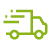FREE Delivery Over £50*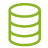ClearPay - Pay in 4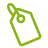Best Price Guarantee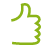Excellent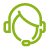Chat to an Expert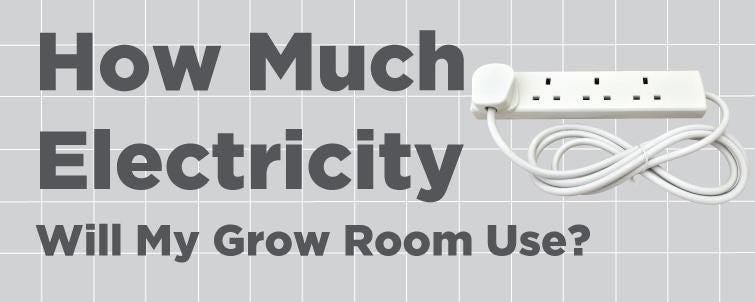# How much electricity will my Grow Room use?

How much is your grow room costing you? Probably less than you think. Here's how to calculate your electricity costs.

## Step 1

First, you need to know how many Watts per hour your appliances are using.

Example
The 200mm (8") RVK 200 fan uses up to 104 Watts per hour

## Step 2

Multiply the Watts per hour, by the number of hours you'll be using your appliance/s for.

Example

200mm (8") RVK200

104W x 24hrs = roughly 2,496W a day

Remember, some appliances use more energy at some times than others.

For instance, EC fans use a lot less energy when slowed down with an EC controller.

For items like these, first estimate how many hours you'll run them for at each wattage. Then calculate how many Watts that is in total per day.

Example

200mm (8") Revolution Vector EC Fan

Running at 976m3/h for 6hrs
82.4W x 6hrs = 494W

Running at 610m3/h for 6hrs
26.7W x 6hrs = 160.2W

Running at 366m3/h for 12hrs
10.8W x 12hrs = 129.6W

494W + 160.2W + 129.6W = roughly 783.8W a day

## Step 3

Repeat step 2 for every appliance you use.

Add their wattages together to know how many watts you typically use per day.

## Step 4

Then, you need to know how much you pay per unit.

Normally, this is on your energy bill (as pence per unit). If it's not, just call your supplier.

1 unit = 1,000W (1kW)

So, divide the cost per unit by 1000 to get your cost per W.

1 unit / 1000 = cost per W

Example

15p per unit = 15p per 1000W

So

15p / 1000 = 0.015p per W

Bear in mind that a lot of suppleirs charge less per unit during the night. You can save a lot of money by running lights at this time.

## Step 5

Multiply your daily wattage (calculated in steps 2 and 3) by your cost per W (calculated in step 4)

Example

783.2W x 0.015p = 11.75p a day